## The result is shown below:

Rabin-Karp algorithm代写 The Rabin-Karp algorithm does not need to compare the pattern string with the characters of the matching string.

### 1.True.    Rabin-Karp algorithm代写

time for merge sorting is related to the size of the data,but not to the size of a single data value. Because the merge sorting is based on the idea of divide and conquer, the final operation is to compare the two numbers, so it is independent of the size of the number.

### 2.The merge sort process is as follows:

 0 1 2 3 4 5 6 7 8 9 10 11 12 0 E A S Y Q U E S T I O N 1 A E S Q Y U E S T I O N 2 A E S Q U Y E S T I N O 3 A E Q S U Y E I N O S T 4 A E E I N O Q S S T U Y

3.The binary search tree is as follows: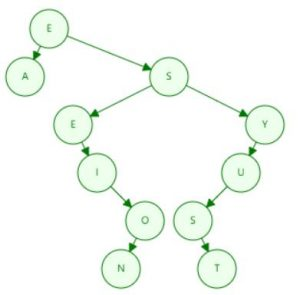4.Missing      Rabin-Karp algorithm代写

5.The result is as follows:

 0 1 2 3 4 5 6 7 8 9 10 11 12 A N Q E S E U S T I Y

### 6.The result is as follows:

 0 1 2 3 4 5 6 7 8 9 10 11 12 N A Y O E Q E S S U T I

7.The Rabin-Karp algorithm does not need to compare the pattern string with the characters of the matching string. It only needs to calculate the hash value of the pattern string and then compare it with the hash value of the substring of the same length in the matching string. This avoids having to re-match each character with the pattern string each time the substring in the matching string moves backwards. Moreover, the hash value of the substring can also be updated within a constant time when the substring moves backward.

8.The BFS search will traverse all child nodes of the current node first, and after traversing all the child nodes, it will traverse the child nodes of the child nodes.

The DFS search will first traverse one of the child nodes of the current node, and then traverse the child nodes of the child node until it traverses to the leaf node and back to the layer above the leaf node.

9.Because the depth of the subtree may be infinite, DFS may never find the correct result, DFS is incomplete. Because DFS finds the “leftmost” solution, regardless of depth or cost, it is not optimal.

### 10.We can use the binary search method to find the number of peaks, so the time complexity is O(logN).    Rabin-Karp algorithm代写

11.The A* algorithm guides the search process by introducing evaluation functions, which are usually much easier to solve than the problem we are trying to solve, but can better estimate the probability of the current correct result.

12.D

13.D

14.A

15.D

16.D

### 17.The result is as follows:

 A B C D Dis ∞ ∞ ∞ ∞ 0 5 3 12 0 5 3 min{12，5+2}=7 0 5 3 min{7，3+3}=6 0 5 3 6

18.C     Rabin-Karp algorithm代写

19.C

20.A

21.The order is as follows:

1. AB C D E F
2. AB D E C F

22.The result is as follows:

### 23.E        Rabin-Karp algorithm代写

24.The result is as follows: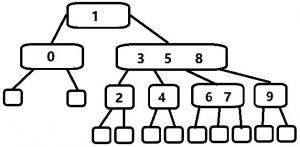25.The result is as follows:

26.D

### 27.Explain as follows:

a)If the optimal solution of a problem contains the optimal solution of the subproblem, we say that the problem has the optimal substructure property.Overlapping subproblems refer to the fact that when solving the problem from top to bottom with recursive algorithm, the subproblems generated each time are not always new problems, and some subproblems will be repeatedly calculated.

b)Memoization is a technique for caching and reusing previously computed results.

c)The objective function means that we need to represent the problem in terms of minimizing (or possibly maximizing) some weight function.

28.Y Because the student number is generally small, when the value is small, the speed time complexity of the cardinal sorting is faster than the general sorting time complexity O(NlogN).

#### 29.The sorting process is as follows:         Rabin-Karp algorithm代写

 KEY d2 d1 d0 KEY d2 d1 d0 COW C O W SEA S E A DOG D O G MOB M O B SEA S E A JOB J O B RUG R U G DOG D O G ROW R O W RUG R U G MOB M O B CAT C A T JOB J O B COW C O W CAT C A T ROW R O W KEY d2 d1 d0 KEY d2 d1 d0 CAT C A T CAT C A T SEA S E A COW C O W MOB M O B DOG D O G JOB J O B JOB J O B DOG D O G MOB M O B COW C O W ROW R O W ROW R O W RUG R U G RUG R U G SEA S E A

30.O(n). If all the keys have the same hash value, then all the keys are equivalent to being stored in a linked list.

31.The perfect hash of theory does not currently exist. Because the range of values for the hash function must be finite, the number of data that needs to be hashed is indeterminate. Assume that the perfect hash function H(x) has a value range of 0~(N-1). If you need to hash (N+1), collision must occur at this time, H(x) is not perfect.

### 32.Process is as follows: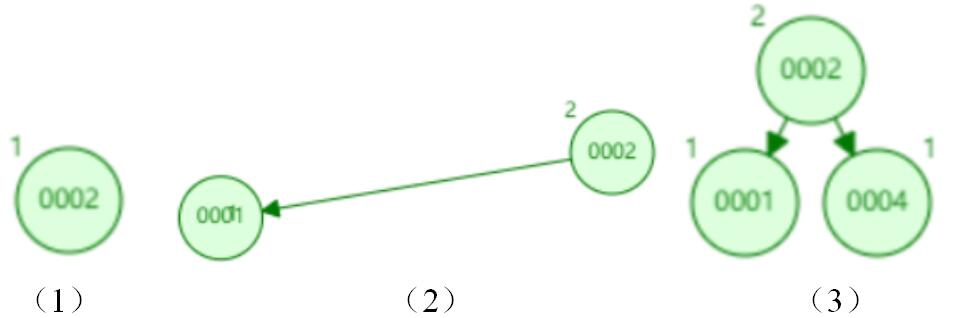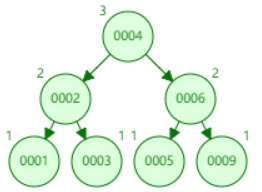（7）

### 33.Result is as follows:       Rabin-Karp algorithm代写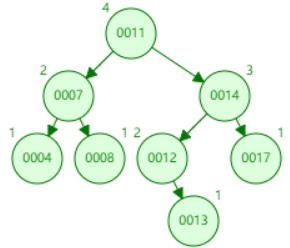34.B

35.C

36.For example, the cost from A to B is 3, the cost from B to C is 4, and the cost from A to C is 7. At this time, the shortest path from A to C is from A to B to C, and the cost is 7. If the cost of each edge is increased by 1, the shortest path from A to C becomes direct from A to C at a cost of 8.

#### 37.Because both algorithms end up with the correct shortest path,          Rabin-Karp algorithm代写

the total weight of the shortest path finally calculated by the two is the same.However, the Dijkstra algorithm is to find the closest point to the source point each time, and the Bellman Ford algorithm performs global update every time, So when there are two shortest paths in a graph and the number of edges is different, the final result of the Dijkstra algorithm is likely to be a path with fewer edges, and the Bellman Ford algorithm tends to have a path with more edges. I can’t make sure that this conclusion is completely correct, but I have found that some examples conform to this rule, which is enough to show that the path calculated by the Dijkstra algorithm and the Bellman Ford algorithm may be different.

38.Using a circular buffer (circular array) to implement a queue can apply for space from the beginning, and the linked list needs to apply for space each time, and the application space will have time overhead.

39.Using a linked list to implement a queue is more flexible in space, because the linked list can apply for space each time it is used, regardless of the initial space limit, and the circular buffer (circular array) is limited by the space of the initial application.

### 40.A 1024-bit number can be represented as a sequence of 16 64-bit integers.        Rabin-Karp algorithm代写

If we add two k-chunk integers this involves calculating k additions, each of b-bit integers. We can add two n-bit integers in k operations. The product x×y involves calculating products of all of the chunks of each number taken in pairs, each product being multiplied by an appropriate power of the base. In general multiplying two n-digit integers together involves: O(n2) multiplications; O(n2) additions.

41.We can use the binary heap or Fibonacci heap to optimize the Dijkstra algorithm. Using a binary heap can optimize the time complexity to O(( E+V )logV). Using the Fibonacci heap can optimize the time complexity to O(E+VlogV).

#### 42.Answer the following:            Rabin-Karp algorithm代写

a)The Fibonacci sequence refers to such a sequence: 1, 1, 2, 3, 5, 8, 13, 21, 34, … mathematically, the Fibonacci sequence is defined by a recursive method as follows :F(1)=1, F(2)=1, F(n)=F(n-1)+F(n-2)(n>=3, n∈N*).

b)The Fibonacci sequence is related to the generation of the rectangular area, from which a property of a Fibonacci sequence can be derived. The sum of the squares of the first few terms of the Fibonacci sequence can be seen as squares of different sizes. Due to the recursive formula of Fibonacci, they can be combined into a large rectangle. Thus the sum of the areas of all the small squares is equal to the area of the large rectangle.

c)In order to speed up the generation of the Fibonacci sequence, we can use the idea of dynamic programming, change the time with space, and record the value of each item calculated, so that only one of the first two values of the item is needed to calculate an item. Can be calculated directly, reducing a lot of double counting.